#Linear Independence and the WronskianLet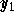and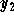be two differentiable functions. The Wronskian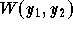, associated toand, is the function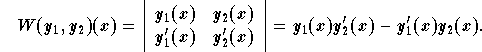We have the following important properties:

(1)
Ifandare two solutions of the equation y'' + p(x)y' + q(x)y = 0, then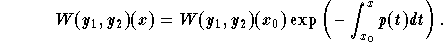(2)
Ifandare two solutions of the equation y'' + p(x)y' + q(x)y = 0, then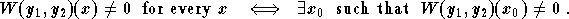In this case, we say thatandare linearly independent.

(3)
Ifandare two linearly independent solutions of the equation y'' + p(x)y' + q(x)y = 0, then any solution y is given by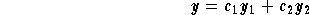for some constant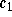and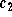. In this case, the set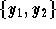is called the fundamental set of solutions.

Example: Letbe the solution to the IVP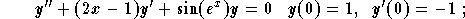andbe the solution to the IVP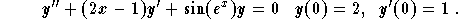Find the Wronskian of. Deduce the general solution to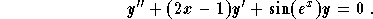Solution: Let us write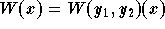. We know from the properties that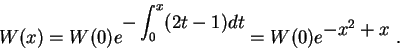Let us evaluate W(0). We have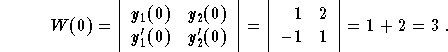Therefore, we have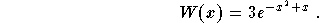Since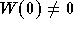, we deduce thatis a fundamental set of solutions. Therefore, the general solution is given by,

where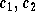are arbitrary constants.[Differential Equations] [First Order D.E.] [Second Order D.E.]
[Geometry] [Algebra] [Trigonometry ]
[Calculus] [Complex Variables] [Matrix Algebra]S.O.S MATHematics home page

Do you need more help? Please post your question on our S.O.S. Mathematics CyberBoard.Author: Mohamed Amine Khamsi